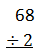# Does the Size of a Fraction Bar Matter?

When we teach children to add, subtract, and multiply, we commonly see a vertical format used in school.

9
+ 6
15

Then, the student learns division. Students usually see the division algorithm represented horizontally.A student may not, beyond a worksheet with dozens of problems, be comfortable with a vertical format for division.It almost feels strange to me to the type the problem vertically. Then comes fractions. We did the following exercise in a workshop I led today. I wanted participants to consider the question, “Does the size of a fraction bar matter?”

Below is an image of the problems we worked on in groups. Calculators were allowed. Spirited discussion and abundant disagreement followed.As the back of the textbook often says, “Answers will vary.” The participants’ responses, split up by problem, appear below.How can we resolve this conflict? Let’s type it into a reliable computation source, like Wolfram Alpha. It depends on how we type the input.Above is one way to type the input. Below is another way to type the input.Why the difference? Because multiplication is commutative, but division is not. 3 x 4 is the same as 4 x 3. But 3 divided by 4 does not yield the same result as 4 divided by 3.

In the original input, where I typed 3/4/1/2, the computer interpreted this as the product of 3 and the multiplicative inverses of 4, 1, and 2, like this:In the second example, we see the computer associates the fractions ¾ and ½, treating the middle fraction bar as a grouping symbol.The confusion for me stems from converting between vertical format and horizontal format. We teach students to follow order of operations, to divide in a horizontal statement as they encounter it from left to right. In school mathematics, we commonly write the fractions vertically when we mean to take the ratio between two ratios, specifically ¾ and ½ in this case.

Here is a state assessment practice item, taken from the Nebraska Department of Education website, that demonstrates this understanding of the vertical format when dividing fractions.Let’s look at this Word document, specifically this problem. The author of the item intends for students to invert 7/3 and multiply. I worry a student may see this problem in a horizontal format asthe work shown to the left, which gives a result which is obviously not an available choice, but if we are examining item reliability, I wonder if we should have the conversation about whether this expression should be typed in the following way:Again, I’m just posing the question about the test item. I want to be careful in how I represent problems with my students. I want my own students to understand the context matters. I want my students to be procedurally fluent with fractions when they solve problems in the world. The conversation in this morning's workshop was great. Participants reflected on how what they say to their students while they teach the students can have unintended consequences on how students view procedures with fractions.

Here’s a link for a great blog entry on this topic:
http://pballew.blogspot.com/2009/01/why-we-flip-and-multiply.html

This site uses Akismet to reduce spam. Learn how your comment data is processed.